#JavaScript 原来可以这么优雅

0
0
01. 云栖社区>
2. 前端那些事儿>
3. 博客>
4. 正文

## JavaScript 原来可以这么优雅

cacao111 2018-07-11 14:51:05 浏览2370

# JavaScript 优雅的实现方式

1、简短优雅地实现 sleep 函数

1.1 普通版

functionsleep(sleepTime) {for(varstart=+newDate;+newDate-start<=sleepTime;) {}}vart1=+newDate()sleep(3000)vart2=+newDate()console.log(t2-t1)

1.2 Promise 版本

functionsleep(time) {returnnewPromise(resolve=>setTimeout(resolve, time))}constt1=+newDate()sleep(3000).then(()=>{constt2=+newDate()console.log(t2-t1)})

1.3 Generator 版本

functionsleep(delay) {returnfunction(cb) {setTimeout(cb.bind(this), delay)  };}function*genSleep() {constt1=+newDate()yieldsleep(3000)constt2=+newDate()console.log(t2-t1)}async(genSleep)functionasync(gen) {constiter=gen()functionnextStep(it) {if(it.done)returnif(typeofit.value==="function") {it.value(function(ret) {nextStep(iter.next(ret))      })    }else{nextStep(iter.next(it.value))    }  }nextStep(iter.next())}

co之所以这么火并不是没有原因的，当然不是仅仅实现sleep这么无聊的事情，而是它活生生的借着generator/yield实现了很类似async/await的效果！这一点真是让我三观尽毁刮目相看。

constco=require("co")functionsleep(delay) {returnfunction(cb) {setTimeout(cb.bind(this), delay)  }}co(function*() {constt1=+newDate()yieldsleep(3000)constt2=+newDate()console.log(t2-t1)})

1.4 Async/Await 版本

functionsleep(delay) {returnnewPromise(reslove=>{setTimeout(reslove, delay)  })}!asyncfunctiontest() {constt1=+newDate()awaitsleep(3000)constt2=+newDate()console.log(t2-t1)}()

Async/Await 比 Generator 的四个改进点

1.5 不要忘了开源的力量

constsleep=require("sleep")constt1=+newDate()sleep.msleep(3000)constt2=+newDate()console.log(t2-t1)

2、获取时间戳

2.1 普通版

vartimestamp=newDate().getTime()

2.2 进阶版

vartimestamp=(newDate()).valueOf()

valueOf 方法返回对象的原始值(Primitive,'Null','Undefined','String','Boolean','Number'五种基本数据类型之一)，可能是字符串、数值或 bool 值等，看具体的对象。

2.3 终极版

vartimestamp=+newDate()

+new Date() 相当于 ToNumber(new Date())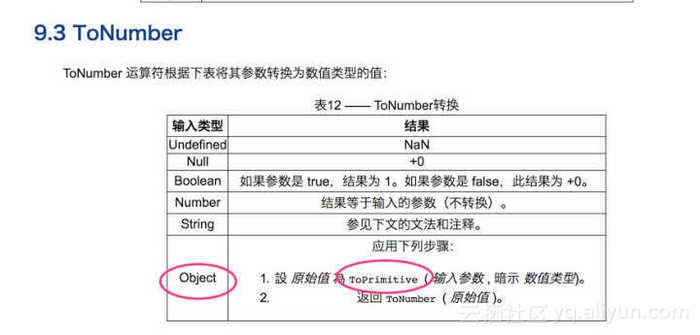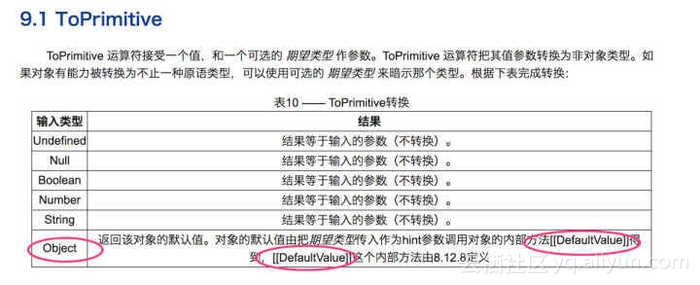ToPrimitive(obj,preferredType)

JavaScript引擎内部转换为原始值ToPrimitive(obj,preferredType)函数接受两个参数，第一个obj为被转换的对象，第二个preferredType为希望转换成的类型（默认为空，接受的值为Number或String）

toString用来返回对象的字符串表示。

varobj={};console.log(obj.toString());//[object Object]vararr2=[];console.log(arr2.toString());//""空字符串vardate=newDate();console.log(date.toString());//Sun Feb 28 2016 13:40:36 GMT+0800 (中国标准时间)

valueOf方法返回对象的原始值，可能是字符串、数值或bool值等，看具体的对象。

varobj={  name:"obj"}console.log(obj.valueOf())//Object {name: "obj"}vararr1=console.log(arr1.valueOf())//vardate=newDate()console.log(date.valueOf())//1456638436303如代码所示，三个不同的对象实例调用valueOf返回不同的数据

var time=new Date();

+time

time是个日期对象，根据ToNumber的转换规则，所以相当于：ToNumber(ToPrimitive(time))

3、数组去重

3.1 普通版

vara=[1,1,'1','2',1]functionunique(arr) {varres=[]for(vari=0, len=arr.length; i

3.2 进阶版

vara=[1,1,'1','2',1]functionunique(arr) {returnarr.filter(function(ele,index,array){returnarray.indexOf(ele)===index//很巧妙,这样筛选一对一的,过滤掉重复的})}console.log(unique(a))//[1, 2, "1"]

3.3 时间复杂度为O(n)

vara=[1,1,'1','2',1]functionunique(arr) {varobj={}returnarr.filter(function(item,index,array){returnobj.hasOwnProperty(typeofitem+item)?false:(obj[typeofitem+item]=true)    })}console.log(unique(a))//[1, 2, "1"]

filter是数组迭代的方法，内部还是一个for循环，所以时间复杂度是O(n)。

3.4 终极版

constunique=a=>[...newSet(a)]

4、数字格式化 1234567890 --> 1,234,567,890

4.1 普通版

functionformatNumber(str) {letarr=[],    count=str.lengthwhile(count>=3) {arr.unshift(str.slice(count-3, count))    count-=3}//如果是不是3的倍数就另外追加到上去str.length%3&&arr.unshift(str.slice(0,str.length%3))returnarr.toString()}console.log(formatNumber("1234567890"))//1,234,567,890

4.2 进阶版

functionformatNumber(str) {//["0", "9", "8", "7", "6", "5", "4", "3", "2", "1"]returnstr.split("").reverse().reduce((prev,next,index)=>{return((index%3)?next:(next+','))+prev  })}console.log(formatNumber("1234567890"))//1,234,567,890

4.3 正则版

functionformatNumber(str) {returnstr.replace(/\B(?=(\d{3})+(?!\d))/g,',')}console.log(formatNumber("123456789"))//1,234,567,890

/\B(?=(\d{3})+(?!\d))/g：正则匹配边界\B，边界后面必须跟着(\d{3})+(?!\d);

(\d{3})+：必须是1个或多个的3个连续数字;

(?!\d)：第2步中的3个数字不允许后面跟着数字;

(\d{3})+(?!\d)：所以匹配的边界后面必须跟着3*n（n>=1）的数字。

4.4 API版

(123456789).toLocaleString('en-US')//1,234,567,890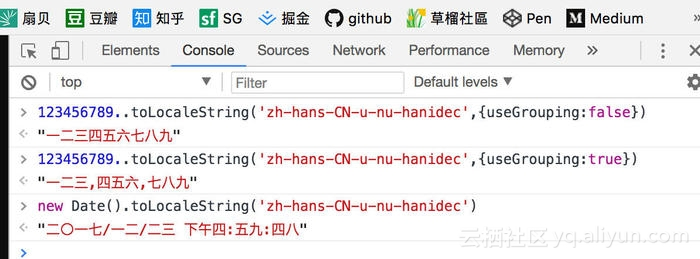Intl 对象是 ECMAScript 国际化 API 的一个命名空间，它提供了精确的字符串对比，数字格式化，日期和时间格式化。Collator，NumberFormat 和 DateTimeFormat 对象的构造函数是 Intl 对象的属性。

newIntl.NumberFormat().format(1234567890)//1,234,567,890

5、交换两个整数

let a = 3,b = 4 变成 a = 4, b = 3

5.1 普通版

leta=3,b=4lettemp=aa=bb=tempconsole.log(a, b)

5.2 进阶版

leta=3,b=4a+=bb=a-ba-=bconsole.log(a, b)

5.3 终极版

leta=3,b=4a^=b  b^=a  a^=bconsole.log(a, b)

a=011(^) b=100则  a=111(a^b的结果赋值给a，a已变成了7)(^) b=100则  b=011(b^a的结果赋给b，b已经变成了3)(^) a=111则  a=100(a^b的结果赋给a，a已经变成了4)

1.对于开始的两个赋值语句，a = a ^ b，b = b ^ a，相当于b = b ^ (a ^ b) = a ^ b ^ b，而b ^ b 显然等于0。因此b = a ^ 0，显然结果为a。

2. 同理可以分析第三个赋值语句，a = a ^ b = (a ^ b) ^ a = b

^ 即”异或“运算符。

^ 运算符的特点是与0异或，保持原值；与本身异或，结果为0。

5.4 究极版

vara=3,b=4;[b, a]=[a, b]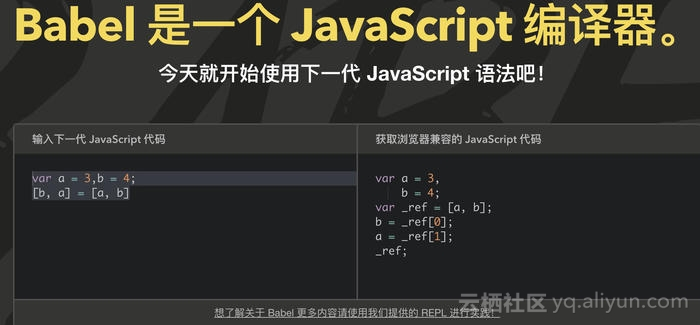5.4 ECMAScript 自动分号;插入(作为补充，防止大家以后踩坑)

JavaScript 不是一个没有分号的语言，恰恰相反上它需要分号来就解析源代码。 因此 JavaScript 解析器在遇到由于缺少分号导致的解析错误时，会自动在源代码中插入分号。

5.4.1例子

varfoo=function() {}//解析错误，分号丢失test()

varfoo=function() {};//没有错误，解析继续test()

5.4.2工作原理

(function(window,undefined) {functiontest(options) {log('testing!')        (options.list||[]).forEach(function(i) {        })options.value.test('long string to pass here','and another long string to pass')return{foo:function() {}        }    }window.test=test})(window)(function(window) {window.someLibrary={}})(window)

(function(window,undefined) {functiontest(options) {//没有插入分号，两行被合并为一行log('testing!')(options.list||[]).forEach(function(i) {        });//<- 插入分号options.value.test('long string to pass here','and another long string to pass');//<- 插入分号return;//<- 插入分号, 改变了 return 表达式的行为{//作为一个代码段处理foo:function() {}        };//<- 插入分号}window.test=test;//<- 插入分号//两行又被合并了})(window)(function(window) {window.someLibrary={};//<- 插入分号})(window);//<- 插入分号

5.4.3 ECMAScript对自动分号插入的规则

**必须用分号终止某些 ECMAScript 语句 ( 空语句 , 变量声明语句 , 表达式语句 , do-while 语句 , continue 语句 , break 语句 , return 语句 ,throw 语句 )。这些分号总是明确的显示在源文本里。然而，为了方便起见，某些情况下这些分号可以在源文本里省略。描述这种情况会说：这种情况下给源代码的 token 流自动插入分号。**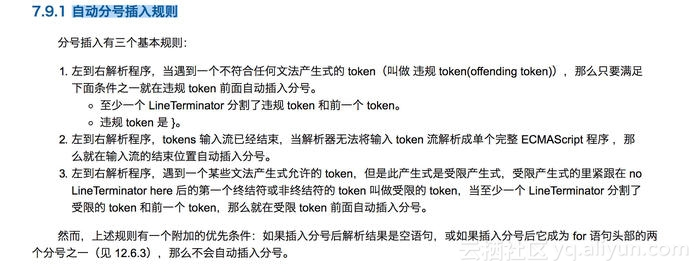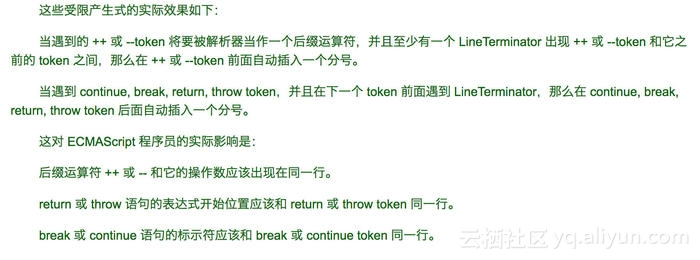5.4.3.1 ASI的规则

1. 新行并入当前行将构成非法语句，自动插入分号。

if(1<10) a=1console.log(a)//等价于if(1<10) a=1;console.log(a);

2. 在continue,return,break,throw后自动插入分号

return{a:1}//等价于return;{a:1};

3. ++、--后缀表达式作为新行的开始，在行首自动插入分号

a++c//等价于a;++c;

4. 代码块的最后一个语句会自动插入分号

function(){ a=1}//等价于function(){ a=1; }

5.4.3.2 No ASI的规则

1. 新行以 ( 开始

vara=1varb=a(a+b).toString()//会被解析为以a+b为入参调用函数a，然后调用函数返回值的toString函数vara=1varb=a(a+b).toString()

2. 新行以 [ 开始

vara=['a1','a2']varb=a[0,1].slice(1)//会被解析先获取a，然后调用a.slice(1)。//由于逗号位于[]内，且不被解析为数组字面量，而被解析为运算符，而逗号运算符会先执行左侧表达式，然后执行右侧表达式并且以右侧表达式的计算结果作为返回值vara=['a1','a2']varb=a[0,1].slice(1)

3. 新行以 / 开始

vara=1varb=a/test/.test(b)///会被解析为整除运算符，而不是正则表达式字面量的起始符号。浏览器中会报test前多了个.号vara=1varb=a/test/.test(b)

4. 新行以 + 、 - 、 % 和 * 开始

vara=2varb=a+a//会解析如下格式vara=2varb=a+a

5. 新行以 , 或 . 开始

vara=2varb=a.toString()console.log(typeofb)//会解析为vara=2varb=a.toString()console.log(typeofb)

//三个空语句;;;//只有if条件语句，语句块为空语句。//可实现unless条件语句的效果if(1>2);elseconsole.log('2 is greater than 1 always!');//只有while条件语句，循环体为空语句。vara=1while(++a<100);

5.4.4 结论

6、将 argruments 对象(类数组)转换成数组

{0:1,1:2,2:3,length:3}这种形式的就属于类数组，就是按照数组下标排序的对象，还有一个length属性，有时候我们需要这种对象能调用数组下的一个方法，这时候就需要把把类数组转化成真正的数组。

6.1 普通版

varmakeArray=function(array) {varret=[]if(array!=null) {vari=array.lengthif(i==null||typeofarray==="string") ret=arrayelsewhile(i) ret[--i]=array[i];  }returnret}makeArray({0:1,1:2,2:3,length:3})//[1,2,3]

6.2 进阶版

vararr=Array.prototype.slice.call(arguments);

The slice() method returns a shallow copy of a portion of an array into a new array object.

slice.call的作用原理就是，利用call，将slice的方法作用于arrayLike，slice的两个参数为空，slice内部解析使得arguments.lengt等于0的时候 相当于处理slice(0)： 即选择整个数组，slice方法内部没有强制判断必须是Array类型，slice返回的是新建的数组（使用循环取值）”，所以这样就实现了类数组到数组的转化，call这个神奇的方法、slice的处理缺一不可。

//This will work for genuine arrays, array-like objects,//NamedNodeMap (attributes, entities, notations),//NodeList (e.g., getElementsByTagName), HTMLCollection (e.g., childNodes),//and will not fail on other DOM objects (as do DOM elements in IE < 9)Array.prototype.slice=function(begin,end) {//IE < 9 gets unhappy with an undefined end argumentend=(typeofend!=='undefined')?end:this.length;//For native Array objects, we use the native slice functionif(Object.prototype.toString.call(this)==='[object Array]'){return_slice.call(this, begin, end);      }//For array like object we handle it ourselves.vari, cloned=[],        size, len=this.length;//Handle negative value for "begin"varstart=begin||0;      start=(start>=0)?start:Math.max(0, len+start);//Handle negative value for "end"varupTo=(typeofend=='number')?Math.min(end, len):len;if(end<0) {        upTo=len+end;      }//Actual expected size of the slicesize=upTo-start;if(size>0) {        cloned=newArray(size);if(this.charAt) {for(i=0; i

6.3 ES6 版本

vararr=Array.from(arguments);

varargs=[...arguments];

ES6中的扩展运算符...也能将某些数据结构转换成数组，这种数据结构必须有便利器接口。

7、数字取整 2.33333 => 2

7.1 普通版

consta=parseInt(2.33333)

parseInt()函数解析一个字符串参数，并返回一个指定基数的整数 (数学系统的基础)。这个估计是直接取整最常用的方法了。

7.2 进阶版

consta=Math.trunc(2.33333)

Math.trunc()方法会将数字的小数部分去掉，只保留整数部分。

Math.trunc=Math.trunc||function(x) {if(isNaN(x)) {returnNaN;  }if(x>0) {returnMath.floor(x);  }returnMath.ceil(x);};

7.3 黑科技版

7.3.1 ~~number

console.log(~~47.11)//-> 47console.log(~~1.9999)//-> 1console.log(~~3)//-> 3console.log(~~[])//-> 0console.log(~~NaN)//-> 0console.log(~~null)//-> 0

//异常情况console.log(~~2147493647.123)//-> -2147473649

7.3.2 number | 0

| (按位或) 对每一对比特位执行或（OR）操作。

console.log(20.15|0);//-> 20console.log((-20.15)|0);//-> -20console.log(3000000000.15|0);//-> -1294967296

7.3.3 number ^ 0

^ (按位异或)，对每一对比特位执行异或（XOR）操作。

console.log(20.15^0);//-> 20console.log((-20.15)^0);//-> -20console.log(3000000000.15^0);//-> -1294967296

7.3.4 number << 0

<< (左移) 操作符会将第一个操作数向左移动指定的位数。向左被移出的位被丢弃，右侧用 0 补充。

console.log(20.15<<0);//-> 20console.log((-20.15)<<0);//-20console.log(3000000000.15<<0);//-> -1294967296

8、数组求和

8.1 普通版

letarr=[1,2,3,4,5]functionsum(arr){letx=0for(leti=0; i

8.2 优雅版

letarr=[1,2,3,4,5]functionsum(arr) {returnarr.reduce((a,b)=>a+b)}sum(arr)//15

8.3 终极版

letarr=[1,2,3,4,5]functionsum(arr) {returneval(arr.join("+"))}sum(arr)//15

eval 不容易调试。用 chromeDev 等调试工具无法打断点调试，所以麻烦的东西也是不推荐使用的…

cacao111
+ 关注